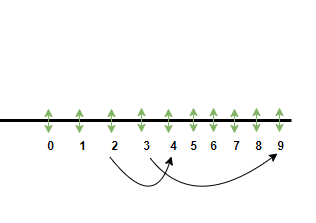GeeksforGeeks App
Open AppBrowser
Continue

# What are the numbers which cannot come to the unit place of a perfect square?

A system was introduced to define the numbers present from negative infinity to positive infinity. The system is known as the Number system. Number system is easily represented on a number line and Integers, whole numbers, natural numbers can be all defined on a number line. The number line contains positive numbers, negative numbers, and zero. Perfect square can be easily found on the number line as well.

### Perfect Square

Perfect squares are defined as the numbers found in the number system that are the squares of numbers belonging to the number system only. For instance, the perfect square of 5 is 25, the perfect square of 7 is 49.Example: Write down the perfect squares of numbers from 1 to 13.

The perfect square of numbers from 1 to 13 are as follows,

### Numbers that cannot come at the unit place of a Perfect square

From the perfect squares found of all the numbers, it is obtained that 2, 3, 7, and 8 are the number that can never exist on the unit place of a Perfect Square. The other numbers like 1, 4, 5, 6, 9 can come at the unit place of the perfect square. Examples of those are, square of 9 is 81, square of 2 is 4, square of 5 is 25, square of 6 is 36, square of 7 is 49, and so on.

Can 0 occur at the unit place of a perfect square?

If there are 0s in pairs at the end of the perfect square, for example, the perfect square of 10 is 100. Hence, in this manner 0s can exist at the unit place of the perfect square.

Question: Which of the following are and are not perfect squares.

25, 112, 64, 144, 38, 36, 78, 81, 100, 50.